# Survival Analysis: Comparison of Two Survival Curves – Rubinstein

Hypothesis: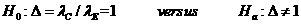Data Input: (Help) (Example)

 Input Results α β δ N MS NC QC NE QE D T0 T-T0

Note:

 Variables Descriptions α Significance level 1-β Power of the test δ Minimum hazards ratio,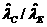, the ratio of two estimated hazard rates MS Control median survival (month) QC Proportion in control group QE Proportion in experimental group T0 Accrual duration (month) T Length of study (month) T-T0 Follow-up duration (month) N Required sample size NC Number of sample size in control group NE Number of sample size in experimental group D Total number of deaths

Help Aids

Application:  The determination of the number of patients needed in a prospective comparison of survival curves with losses to follow-up, when the control group patients have already been followed for some period, following hypotheses are usually used:where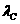is the hazard rate of patients in control group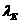is the hazard rate of patients in experimental group

Procedure:

1. Enter

a)   value of α, the probability of type I error

b)   value of β, the probability of type II error, or (1-power) of the test

c)   value of δ, the minimum hazards ratio, it is specified in alternate hypothesis

d)   value of MS, the control median survival (month), it is estimated from existing control data

e)   value of QC, the proportion of patient in control group compared with experimental group

f)   value of QE, the proportion of patient in experimental group compared with control group, where QC + QE = 1

h)   value of T0, accrual duration (month), the length of time to recruit patients for study

g)   value of T-T0, follow-up duration (month, the length of study time of all recruited patients to the end of study T

1. Click the button “Calculate” to obtain

a)    value of N, the total sample size of patients in the control and experimental arm

b)    value of NC, the sample size of patients in the control arm

c)    value of NE, the sample size of patients in the experimental arm

d)    value of D, the total number of deaths at the end of study

Assumption:

1.      During the accrual period of T years, patients enter trial according to a Poisson process with rate n per year. They are randomized with equal probability to either the control or experimental treatment.

2.      The times from entry to death are independent with common exponential distribution within each treatment. The median time to death for the control treatment is MC and from a standard result for the exponential distribution, the death hazard rate is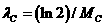.

3.      The times from entry to loss to follow-up are independent of each other and of the entry times and death times. They have exponential distributions with exponential loss to follow-up rates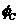for the control group and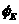for the experimental group.

Theory:

We wish to teat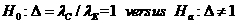at significance level α and to have power 1-β against the alternative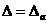for prespecified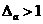.

Let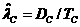,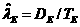and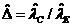where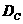and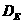are observed numbers of deaths and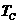and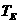are observed total time at risk (person-years exposure) for the control and treatment groups respectively.

Distribution of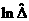is used because it is less skewed and have a more accurate asymptotic approximation.is asymptotically normally distributed with mean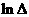and variance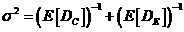, where E donotes expectation.

Under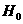, the asymptotic size α test of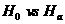rejects for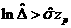, where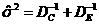and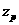is the standard normal (1- p) quantile.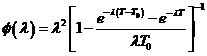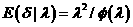The sample size to achieve specified significance level and power is, whereis the upper 100(1-p) percentile of the standard normal distribution.

The above equation can be changed to two-sided tests by changing the above α to α/2. Here the calculator we use two-sided test.

Notations:

α:         The probability of type I error (significance level) is the probability of rejecting the true null hypothesis.

β:         The probability of type II error (1 – power of the test) is the probability of not rejecting the false null hypothesis.

Example:

Consider that a clinical trial is to be conducted for a disease with moderate levels of mortality with hazard rare λ=1.22, yielding 50% survivors after 0.57 month (MS=0.57). Suppose that with treatment we are interested in a reduction in hazard rate (δ=1.3). With equal-sized group (QC=QE=0.5), a two sided significance level test (α =0.05) with power 80% power (β=0.2), and assume that recruitment was to be terminated after 4 years of a 5-year study (T=5, T0=4, T-T0=1), then the required sample size is approximate 498 (N=498) and the total number of deaths is 264 (ND=458).

Reference:

1. Rubinstein, Gail, Santner (1981) J Chron Dis 8: 67-74
2. Rubinstein, Gail, Santner (1981) J Chron Dis 34: 469-479